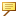Example: Including Units with Relations
This example uses the following parameters and dimensions:
Parameter or dimension
Units
A
unitless
B
inch
d1
mm
d2
mm
The following table lists sample relations that use parameters with and without units.You can declare units on the left side of a relation only for newly created parameters.
Sample Relation
Verification status
d1=d2
OK
d1=d2*A
OK
d1=B
OK
Value of B is converted to mm. For example, if d2=1 and B=1, d1=25.4.
d1=d2+B
OK
d1=5
Error
This relation is not treated as an error if relation_num_const_units is set to no.
d1=5[mm]
OK
A=d1
Error
This relation is not treated as an error if relation_num_const_units is set to no.
A[mm]=d1
Error
A is a unitless parameter and cannot accept units on the left side of the equation.
d1=A
Error
This relation is not treated as an error if relation_num_const_units is set to no.
d1=A[mm]
OK
d1=A[inch]
OK
A is converted to mm. If A=1, then d1=25.4.
d1=d2+5
Error
This relation is not treated as an error if relation_num_const_units is set to no.
d1=d2+5[mm]+.2[cm]
OK
Units are converted to mm.
A=d1+d2
This relation is not treated as an error if relation_num_const_units is set to no.